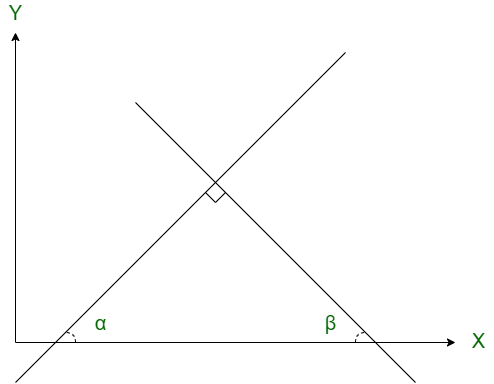GATE | GATE CS 2020 | Question 9

• Last Updated : 12 Jul, 2021

Two straight lines are drawn perpendicular to each other in X−Y plane. If α and β are the acute angles the straight lines make with the X- axis, then α+β is ________ .
(A) 60°
(B) 90°
(C) 120°
(D) 180°

Explanation: According to given question,Now, as we know that, in a triangle sum of internal angles is equal to 180°.
That is,

→ ∠a + ∠b + ∠c = 180°
→ α + β + 90° = 180°
→ α + β = 180° - 90° = 90°
→ α + β = 90°

Option (B) is correct.

Quiz of this Question

My Personal Notes arrow_drop_up